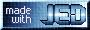# Loving ISIS - Confessions of a Former XSPEC User

Fluxes

A sad truth about X-ray astronomy is that the instrumental effects of the detector should never be ignored. It is very difficult to determine a source's flux to better than order of 10% without reference to an underlying fitted spectral model. (Even then, the accuracy of the flux measurement will be limited by the calibration of the instrument, and the quality of the data.) Still, we like to try.

To that end, I have written several functions that calculate the absorbed X-ray flux in a specified energy band. (Don't even get me started on estimating unabsorbed X-ray fluxes - I will leave the discussion of X-ray absorption to my more knowledgeable German colleagues). One of the functions relies solely upon the spectral data (`data_flux()`), and two rely upon spectral fits to the data (`model_flux()`, `calc_flux()`). Two (`data_flux()`, `model_flux()`) will only operate over the energy bounds within the range of those of the data and model grids (the latter is determined by the internal resolution of the ARF, which is likely different than the PHA grid of the data), and one (`calc_flux()`) will operate over any user-defined grid. These functions are used as follows:

`(pflux, pflux_err, eflux, eflux_err) = data_flux(id,lo,hi);`
This returns the photon and energy flux for dataset `id` between (default: keV energy) bounds given by `lo` and `hi`, plus their estimated errors, as calculated solely from the data and information from the instrumental responses. Since we are "unfolding" the data, this will be subject to some degree of instrument and spectrum-dependent uncertainty. For soft spectra measured by RXTE, this will be large. For 1-7 keV data measured with the Chandra gratings, this will probably be OK. "Unfolding" is dangerous, so be very careful when estimating X-ray fluxes in the absence of a fitted model.
`(pflux, eflux) = model_flux(id,lo,hi);`
This returns the photon and energy flux for dataset `id` between (default: keV energy) bounds given by `lo` and `hi` as calculated from the last spectral model applied to the data. Since it is using an already performed model evaluation, it can only calculate the flux based upon the existing response matrix grids, and therefore cannot calculate fluxes outside of the spectral ranges covered by the responses. The estimated flux, of course, will only be as good as your model fits and as good as the knowledge of the calibrations of the responses.
`(pflux, eflux) = calc_flux(id, bin_lo, bin_hi);`
This returns the photon and energy flux for dataset `id` evaluated over the (default: keV energy) grid defined by `bin_lo` and `bin_hi`, using the defined spectral model. As a full energy grid is being input, fluxes for any range of energies supported by the fit model can be estimated. (Whether the model outside the bounds of the data set grids has any meaning, well, that's another story....) Again, the estimated flux will only be as good as your model fits.

Equivalent Widths

Astronomers often like to quote the strength (or depth, in case of absorption) of a line feature relative to the continuum level in the absence of that feature. To that end, the equivalent width is often used. ISIS does not have an intrinsically defined equivalent width function; however, I have written one (`eqw`) that behaves similar to the XSPEC function. It is slightly more general, however, as it can be applied to any defined line function so long as some parameter (not necessarily called `norm`) sets the line amplitude to zero. The function usage message follows:

``` (ew_ma, ew_ev) = eqw(indx, par [; pmin=#, bounds={val,val}, unit=string, noeval, print]);

Calculate the equivalent width for a model component, in milli-angstrom and eV.

Inputs:
indx  = Data set index to which the model is applied
par   = Number for, *or* string with the name of, the *normalization*
parameter for the model component for which the EW will be calculated

Optional Qualifier Inputs:
pmin  = For parameter par, the value to which it should be set when calculating
the continuum flux without the line (default = 0, but this allows
other parameter toggles to be used).
bounds= Range over which to restrict the  evaluation of the equivalent width.
(Otherwise, uses the full energy range of the arf; see get_model_flux();)
unit  = Units (case insensitive string) for the values of bounds (default="kev")
noeval- If exists, don't do the final eval_counts (parameters will be reset
to initial values, but not evaluated - i.e., plots won't show
the fit with the line, and may otherwise be screwy)
print - If exists, don't output the results, rather, print to screen

Outputs:
ew_ma, ew_ev : Equivalent widths in milli-angstrom and eV
```

Next up: Plotting.

This page was last updated Oct 7, 2013 by Michael Nowak. To comment on it or the material presented here, send email to mnowak@space.mit.edu.